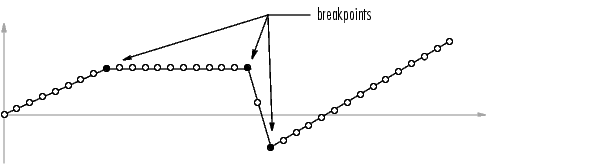MATLAB Function Referencedetrend

Remove linear trends.

Syntax

• y = detrend(x)
y = detrend(x,'constant')
y = detrend(x,'linear',bp)

Description

detrend removes the mean value or linear trend from a vector or matrix, usually for FFT processing.

y = detrend(x) removes the best straight-line fit from vector x and returns it in y. If x is a matrix, detrend removes the trend from each column.

y = detrend(x,'constant') removes the mean value from vector x or, if x is a matrix, from each column of the matrix.

y = detrend(x,'linear',bp) removes a continuous, piecewise linear trend from vector x or, if x is a matrix, from each column of the matrix. Vector bp contains the indices of the breakpoints between adjacent linear segments. The breakpoint between two segments is defined as the data point that the two segments share.detrend(x,'linear'), with no breakpoint vector specified, is the same as detrend(x).

Example

• sig = [0 1 -2 1 0 1 -2 1 0];      % signal with no linear trend
trend = [0 1 2 3 4 3 2 1 0];      % two-segment linear trend
x = sig+trend;                    % signal with added trend
y = detrend(x,'linear',5)         % breakpoint at 5th element

y =

-0.0000
1.0000
-2.0000
1.0000
0.0000
1.0000
-2.0000
1.0000
-0.0000

Note that the breakpoint is specified to be the fifth element, which is the data point shared by the two segments.

Algorithm

detrend computes the least-squares fit of a straight line (or composite line for piecewise linear trends) to the data and subtracts the resulting function from the data. To obtain the equation of the straight-line fit, use polyfit.